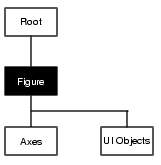MATLAB Function Referencefigure

Create a figure graphics object

Syntax

• ```figure
figure('PropertyName',PropertyValue,...)
figure(h)
h = figure(...)
```

Description

`figure` creates figure graphics objects. Figure objects are the individual windows on the screen in which MATLAB displays graphical output.

```figure ``` creates a new figure object using default property values.

```figure('PropertyName',PropertyValue,...) ``` creates a new figure object using the values of the properties specified. MATLAB uses default values for any properties that you do not explicitly define as arguments.

```figure(h) ``` does one of two things, depending on whether or not a figure with handle `h` exists. If `h` is the handle to an existing figure, `figure(h)` makes the figure identified by `h` the current figure, makes it visible, and raises it above all other figures on the screen. The current figure is the target for graphics output. If `h` is not the handle to an existing figure, but is an integer, `figure(h)` creates a figure and assigns it the handle `h`. `figure(h)` where `h` is not the handle to a figure, and is not an integer, is an error.

```h = figure(...) ``` returns the handle to the figure object.

Remarks

To create a figure object, MATLAB creates a new window whose characteristics are controlled by default figure properties (both factory installed and user defined) and properties specified as arguments. See the properties section for a description of these properties.

You can specify properties as property name/property value pairs, structure arrays, and cell arrays (see the `set` and `get` reference pages for examples of how to specify these data types).

Use `set` to modify the properties of an existing figure or `get` to query the current values of figure properties.

The `gcf` command returns the handle to the current figure and is useful as an argument to the `set` and `get` commands.

Figures can be docked in the desktop. The `Dockable` property determines whether you can dock the figure.

Example

To create a figure window that is one quarter the size of your screen and is positioned in the upper left corner, use the root object's `ScreenSize` property to determine the size. `ScreenSize` is a four-element vector: `[``left``, ``bottom``, ``width``, ``height``]`:

• ```scrsz = get(0,'ScreenSize');
figure('Position',[1 scrsz(4)/2 scrsz(3)/2 scrsz(4)/2])
```

Object HierarchySetting Default Properties

You can set default figure properties only on the root level.

• ```set(0,'DefaultFigureProperty',PropertyValue...)
```

where `Property` is the name of the figure property and `PropertyValue` is the value you are specifying. Use `set` and `get` to access figure properties.

See Also

`axes`, `uicontrol`, `uimenu`, `close`, `clf`, `gcf`, `rootobject`

Object Creation Functions for related functions

Figure Properties descriptions of all figure properties

See Figure Properties in the user guide for more information on figures.figflag Figure Properties© 1994-2005 The MathWorks, Inc.